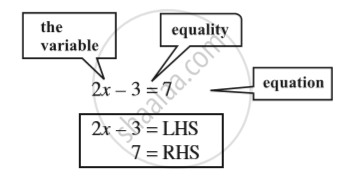Share

# Introduction of Linear Equation

#### definition

An equation between two variables that gives a straight line when plotted on a graph is called linear equation.

#### notes

You have come across several algebraic expressions and equations.
Expression is a mathematical phrase with numbers , variables & operators.
Equation is a statement of equality of two expressions.
For example :
These are linear expressions:
2x, 2x + 1, 3y – 7, 12 – 5z, 5/4 (x-4) +10
These are not linear expressions:
x^2 + 1, y + y^2, 1 + z + z^2 + z^3  (since highest power of variable > 1).
we know that :1) An algebraic equation is an equality involving variables. It  has an equality sign. The expression on the left of the equality sign is the Left Hand Side (LHS). The expression on the right of the equality sign is the Right Hand Side (RHS).

2)  In an equation the values of the expressions on the LHS and RHS are equal. This happens to be true only for certain values of the variable. These values are the solutions of the equation.

3) How to find the solution of an equation?
We assume that the two sides of the equation are balanced. We perform the same mathematical operations on both sides of the equation, so that the balance is not disturbed. A few such steps give the solution.

### Shaalaa.com

Introduction of Linear Equation in One Variable [00:02:12]
S
0%

S